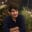Related Tags

general
communitycreator

# What is automata theory?Muhammad Adan

Automata theory is a theoretical branch of computer science. It studies abstract mathematical machines called automatons. When given a finite set of inputs, these automatons automatically imitate humans performing tasks by going through a finite sequence of states.

Through these automatons, mathematicians and computer scientists can understand how machines function, solve problem, learn and what it means for a function to be defined as computable. Along with this, we can also understand how discrete systems behave given a certain set of inputs.

Automatons can be divided into four main families:

1. Finite-State Machine
2. Push-down Automata
3. Linear-bounded Automata
4. Turing Machine

A Finite-State machine is the simplest automata, while a Turing machine is the most complex. A Turing machine is also a Finite-State machine, but the inverse may not be true.

## Notation

An automaton has three basic components:

1. A finite set of inputs I ${x_1, x_2, .... x_n}$
2. A finite set of outputs Z $y_1, y_2, ... y_n$
3. A finite set of states Q whose definition depends on the type of automaton being used.
A Finite-State Machine for a coin-pusher

## Why automatons?

We use automatons instead of computers to model machine behavior because automatons are easier to build, require no hardware, and can be represented by simple mathematical notation.

Automations are abstract machines that do not physically exist.

A computer, on the other hand, is a highly complex machine that is difficult to understand and needs its hardware changed for each different use-case.

RELATED TAGS

general
communitycreator

CONTRIBUTORMuhammad Adan
RELATED COURSES

View all Courses

Keep Exploring

Learn in-demand tech skills in half the time